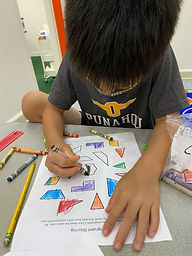## Ms. Brianne

### Target 1​

###### Lesson Type:

Continuation

Number Operation

:

Computation

Subtract within 1,000 (regrouping limited to only one place-value).

###### 1:

Understand why the standard algorithm for subtraction is based on place-value.

###### 2:

Understand that when subtracting a multi-digit number, each place-value is being subtracted individually to create the new number (ones are subtracted from ones, tens are subtracted from tens, etc.)

###### 3:

Understand that when adding a multi-digit number, to solve start in the lowest place-value and go left.

###### 4:

Understand how to regroup based on place-value understandings (i.e., ten ones can be composed into one ten – ten tens can be composed into one hundred).

###### 5:

Understand that subtraction equations can be written both vertically and horizontally.

2nd

###### Vocabulary:

Subtract, Regroup, Tens, Ones

Activities:

*Students solved two-digit subtraction problems involving regrouping. They practiced recording regrouping by crossing out and writing the resulting numbers after trading one ten for ten ones.

*Students played a game where they solved subtraction problems to collect candies on the gameboard.from the trade.### Home Exploration

###### Guiding Questions:## Absent Students:

### Target 2

:

###### 1:

Understand congruence is a geometric word that indicates something is the same shape and size.

2nd

###### Vocabulary:

Congruent, Same

Activities:

*Students were given a variety of shapes or objects for them to demonstrate examples of congruent or not congruent shapes.

*Given a picture of two figures, students stated if the shapes were congruent or not congruent and sorted the picture accordingly.

*Students identified and colored in congruent shapes.### Home Exploration

###### Guiding Questions:### Target 3

:

###### Vocabulary:

Activities:### Home Exploration